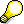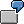Periodic Formulas in Cost Center PlanningUse

In cost center planning, various data cells are linked together using periodic formulas, such as:

Fixed costs + variable costs = overall costs.

are linked together. In the SAP R/3 System, the variables of these formulas correspond to the key figures (or attributes) to be planned, or to the key figure values determined by the system. If you enter one or more key figure values, the system uses heuristic rules to determine which values are to be calculated. If you manually enter all of the values linked by a periodic formula, the system overwrites one of the manually entered values according to a priority list.The periodic formulas are not calculated among the cumulated values of the overview screen, but always by period.

The system applies the periodic formulas in the following ways:

• Individually
• Building on one anotherEnter the values in the object currency for the key figures Variable costs and Fixed costs in the activity-dependent primary cost planning. The system calculates the value for the overall costs according to the formula:

Fixed costs + variable costs = overall costs.

This value is given in the object currency.

Independently of the structure of the planning layout, the costs from the example mentioned above are also translated through further process steps into the controlling area currency and the transaction currency.

Features

Here is a selection of the periodic formulas offered by the system for the planning of CO objects:

• Fixed + variable = Overall (only activity-dependent)
• Fixed = Overall (activity-independent in Cost Center Accounting)
• Costs = price/price unit * plan activity quantity
• Fixed costs/variable costs = Fixed consumption/variable consumption
• Currency translations
• Fixed controlling area currency <-> Fixed object currency
• Variable controlling area currency <-> Variable object currency
• Fixed controlling area currency <-> Fixed transaction currency
• Variable controlling area currency <-> Variable transaction currency
• Fixed controlling area currency <-> Fixed user-definable currency
• Variable controlling area currency <-> Variable user-definable currency
• Overall controlling area currency <-> Overall user-definable currency

Depending on the values you entered during planning, the system calculates the missing values by solving the formulas according to the given variables.

Fixed + Variable = Overall (Primary Costs and Quantities)

This formula is valid for cost element planning and price planning. During the calculations, the system keeps to the following priorities:

1. Fixed + variable = overall

If you have entered a fixed value, as well as a variable and overall value, the system overwrites your overall value.You plan the following primary costs:

Fixed \$20

Variable \$40

Overall \$80

The system calculates an overall value of \$60 and overwrites the value of \$80 in line with the first priority.

2. Overall - variable = fixed

3. Overall - fixed = variable

Fixed Costs = Overall Costs/ Fixed Quantities = Overall Quantities

(Activity-Independent in Cost Center Accounting)

The system uses this formula if you are not planning any activity-dependent costs. If only overall costs exist, the system updates this value as fixed costs.

Overall costs = overall price/price unit * overall quantity

Variable costs = variable price/price unit * variable quantity

The system uses this formula when you plan activity-dependent secondary costs. If you have entered manual prices in activity type planning, or have carried out price calculation, the system uses these values in the activity input planning for valuation of the plan consumption

If you have not planned any prices, you cannot carry out a valuation during secondary planning. Only after price calculation or manual price planning does the system execute a recalculation of the received activity quantities using the prices.Enter the following values in the activity type planning:

Activity quantity of the fixed activity type Drills. 100 hrs

Activity quantity of the variable activity type Drills. 200 hrs

Fixed price \$10/hr

Variable price: \$20/hr

Price unit 1, that is, the price is valid for 1 hour of activity type Drills.

From this, the system calculates the following costs for the receiver of the activity.

 Overall plan costs = Overall price*Total quantity = (Fixed price + variable price) *(Fixed qty + Variable qty) = (101 + 20/1) * (100 + 200) = \$9000
 Plan variable costs = Variable price: * Variable qty = 20/1 * 200 = \$4000
 Fixed plan costs = Overall plan costs – Plan variable costs = \$9000 – \$4000 = \$5000

Fixed Costs/Variable Costs = Fixed Consumption/Variable Consumption

The system uses this formula when you manually plan primary costs. If you have manually planned all four values, and the system determines a breach of this formula due to your entry values, it overwrites the entry value according to the following priorities:

1. Fixed consumption = fixed costs * variable consumption/variable costs

From your entry values, the system first determines the fixed consumption and then overwrites the manually entered value.

2. Variable consumption = variable costs * fixed consumption/fixed costsYou plan the following:

Primary cost planning:

Fixed costs \$2000

Variable costs: \$3000

Activity type planning:

Fixed quantity: 100 hrs

Variable qty: 300 hrs

The system carries out the following calculations:

\$2000 / \$3000 = 100 hrs / 300 hrs

As you have planned all the values manually and the relationships in the formula are not identical, the system overwrites the Fixed consumption value with

Fixed consumption = \$2000 * 300 hrs / \$3000 = 200 hrs

With the value 200 hrs for the fixed consumption, the formula is once again correct.

Currency Translations

During manual planning, the system executes any necessary currency translations if these have been activated in the controlling area. Generally, the system carries out manual planning in the controlling area currency. Additionally, however, you can also use plan values in transaction, object or a freely-definable currency (see also: Currencies in Planning). To do this, you need to add another column to the corresponding planning layout for each additional leading amount in a different currency. The following translations are possible:

Controlling area currency to transaction currency

Controlling area currency to object currency

Controlling area currency to user-definable currency

Transaction currency to controlling area currency

Object currency to controlling area currency

User-definable currency to controlling area currency